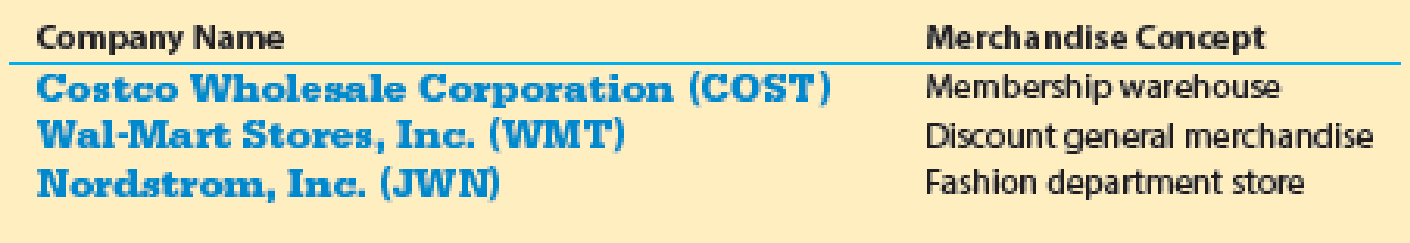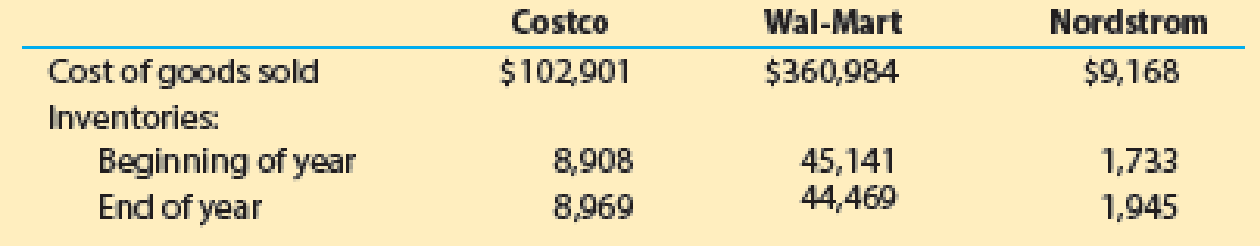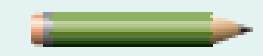# The general merchandise retail industry has a number of segments represented by the following companies: For a recent year, the following cost of goods sold and beginning and ending inventories are provided from corporate annual reports (in millions) for these three companies: a. Determine the inventory turnover ratio for all three companies. Round all calculations to one decimal place. b. Determine the number of days’ sales in inventory for all three companies. Use 365 days and round all calculations to one decimal place. c. Interpret these results based on each company’s merchandising concept.### Financial And Managerial Accounting

15th Edition
WARREN + 1 other
Publisher: Cengage Learning,
ISBN: 9781337902663

#### Solutions

Chapter
Section### Financial And Managerial Accounting

15th Edition
WARREN + 1 other
Publisher: Cengage Learning,
ISBN: 9781337902663
Textbook Problem
9 views

## The general merchandise retail industry has a number of segments represented by the following companies:For a recent year, the following cost of goods sold and beginning and ending inventories are provided from corporate annual reports (in millions) for these three companies:a. Determine the inventory turnover ratio for all three companies. Round all calculations to one decimal place. b. Determine the number of days’ sales in inventory for all three companies. Use 365 days and round all calculations to one decimal place. c.Interpret these results based on each company’s merchandising concept.

(a)

To determine

Determine the inventory turnover for Company C, Company W and Company N.

### Explanation of Solution

Inventory turnover ratio: Inventory turnover ratio is used to determine the number of times inventory used or sold during the particular accounting period. The formula to calculate the inventory turnover ratio is as follows:

Inventory turnover=Cost of goods soldAverage inventory

The inventory turnover ratio for Company C is calculated is calculated as follows:

Inventory turnover=Cost of goods soldAverage inventory=$102,9018,938.5(1)=11.5 Times The inventory turnover ratio for Company W is calculated is calculated as follows: Inventory turnover=Cost of goods soldAverage inventory=$360,98444,805.0(2)=8.1 Times

The inventory turnover ratio for Company N is calculated is calculated as follows:

Inventory turnover=Cost of goods soldAverage inventory=\$9,1681,839(3)=5

b.

To determine

Compute the number of days’ sales in inventory for Company C, Company W and Company N.

(c)

To determine

Interpret the above calculated ratios.

### Still sussing out bartleby?

Check out a sample textbook solution.

See a sample solution

#### The Solution to Your Study Problems

Bartleby provides explanations to thousands of textbook problems written by our experts, many with advanced degrees!

Get Started